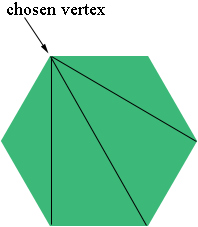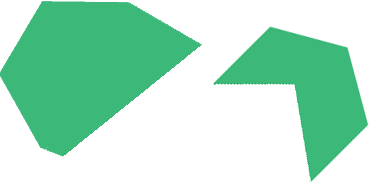SEARCH HOMEMath Central Quandaries & QueriesQuestion from Sandra, a parent: One of my son's math questions is as follows: Suppose I have a shape with 10 sides. I choose a vertex then draw lines to the other vertices that don't share sides with the first vertex. How many vertices will that be and why?Sandra,

I'm going to try this with 6 sides rather than 10. I think the teachers is looking for a polygon, that is a closed figure made up of line segments. I'm going to start with a regular 6-sided polygon, one where all the sides are the same length and all the angles have the same measure. I drew one, chose a vertex and drew lines from my chosen vertex to other vertices that don't share sides with my chosen vertex.I drew 3 lines.

Choose a different vertex. Do you still get 3 lines? What is the polygon is not regular?Choose a vertex and draw the lines. Do you still get 3 lines?

Now try a shape with 10 sides.

PennyMath Central is supported by the University of Regina and The Pacific Institute for the Mathematical Sciences.